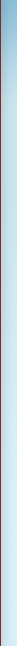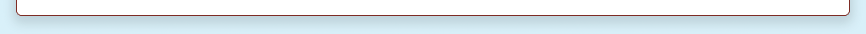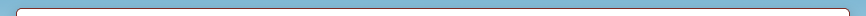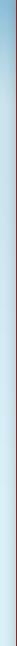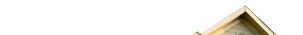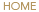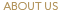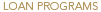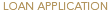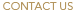Blended Interest Rate
Use this form to calculate your blended interest rate, the average interest rate of a previous rate and a new rate.

 Blended Interest Rate Calculator First Lien Amount \$ First Lien Interest Rate % Second Lien Amount \$ Second Lien Interest Rate % Total Loan Amount \$ Blended Interest Rate Calculation Blended Interest Rate 3.750 %Other Calculators

Payment Calculator
Calculate Payments on a traditional mortgage.

Interest Only Payment Calculator
Calculate your payments with an interest only loan.

2nd Mortgage Calculator

Affordability Calculator
How much house / monthly payment can you afford?

Mortgage Amortization Calculator
Detailed breakdown of principal and interest payments over the life of your loan.

Option ARM Calculator
Calculate four monthly payment options with an adjustable interest rate.

Should I Refinance Calculator
Calculate savings in switching to a new loan.

HELOC Calculator
Calculate payments, interest, and savings for a HELOC.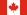Canadian Mortgage Calculator
Calculate Payments on a traditional mortgage.

Commercial Calculators

Max Loan Amount Calculator
Calculate the max loan amount that a property will qualify for.

Debt Service Calculator
Calculate the Debt Service Coverage Ratio (D SCR) of a proposed loan amount.

The accuracy and applicability of the calculators on this site is not guaranteed. The calculation tools available are for demonstration purposes and may not compare directly to your actual loan.